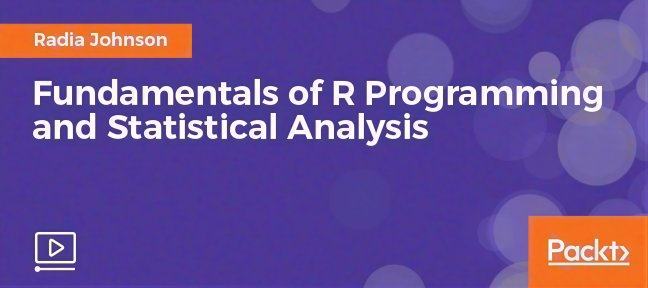# Video Tutorial Fundamentals of R Programming and Statistical Analysiswith R, Data Science

• Course duration : 6h46m
• 30 days money back guaranteeA comprehensive guide to working on statistical data with the R language

• Perform basic and advanced scientific computing.
• Look into the various phases of statistical analysis using R
• Get a clear understanding of how to manipulate data by working on real-world, practical examples

### Course In Detail

The R language is widely used among statisticians and data miners to develop statistical software and data analysis.

In this video course, we’ll start by diving into the different types of R data structures and you’ll learn how the R programming language handles data. Then we’ll look in-depth at manipulating different datasets in R. After that, we’ll dive into data visualization with R, using basic plots, heat maps, and networks. We’ll explore the different flow control loops of the R programming language, and you’ll learn how to debug your code.

In the second half of the course, you’ll get hands-on working with the various statistical methods in R programming. You’ll find out how to work with different probability distributions, various types of hypothesis testing, and statistical analysis with the R programming language.

By the end of this video course, you will be well-versed in the basics of R programming and the various concepts of statistical data analysis with R.

• R Data Structures
• The Course Overview free 00:04:35
• Working with Vectors 00:12:07
• Working with Lists and Attributes 00:13:27
• Working with Multidimensional Arrays and Matrices 00:12:58
• Working with Data Frames and Factors 00:24:51
• Manipulating Datasets with R
• Working with the Subset() and with() Functions 00:10:15
• Working with the which() and grep() Functions 00:14:02
• Working with the sort() and order() Functions 00:11:33
• Working with sapply() and lapply() 00:10:52
• Working with tapply() and table() Functions 00:11:38
• Visualizing Data in R
• Basic Plots in R 00:16:53
• Basic Plots with the ggplot2 Package 00:17:24
• Visualizing Heatmaps 00:21:56
• Visualizing Networks 00:12:34
• Other Visualization Methods 00:09:31
• Flow Control and Debugging Tools
• For Loops Versus the apply() Function 00:16:18
• The if Statement and ifelse() Function 00:14:38
• While and repeat Loops and the Break Statement 00:13:41
• Writing Your Own Functions 00:19:37
• General Programming and Debugging Tools 00:21:05
• Evaluating Probability Distributions
• Descriptive Statistics 00:07:46
• Overview of Probability Distributions 00:13:44
• Fitting Probability Distribution 00:14:39
• Other Statistical Tests to Fit Distributions 00:07:48
• Hypothesis Testing and Statistical Models
• Model Formulas 00:11:33
• One and Two Sample Tests 00:09:19
• Linear Regression 00:10:34
• Analysis of Variance 00:10:26
• Linear Models for Gene Expression Data 00:14:00

## Instructor : Packt

Packt has published 45 tutorials and has sold 10 coursess. See others courses from Packt

• With which software version is this tutorial compatible with?
R
• What is the required level to follow this tutorial ?
beginner